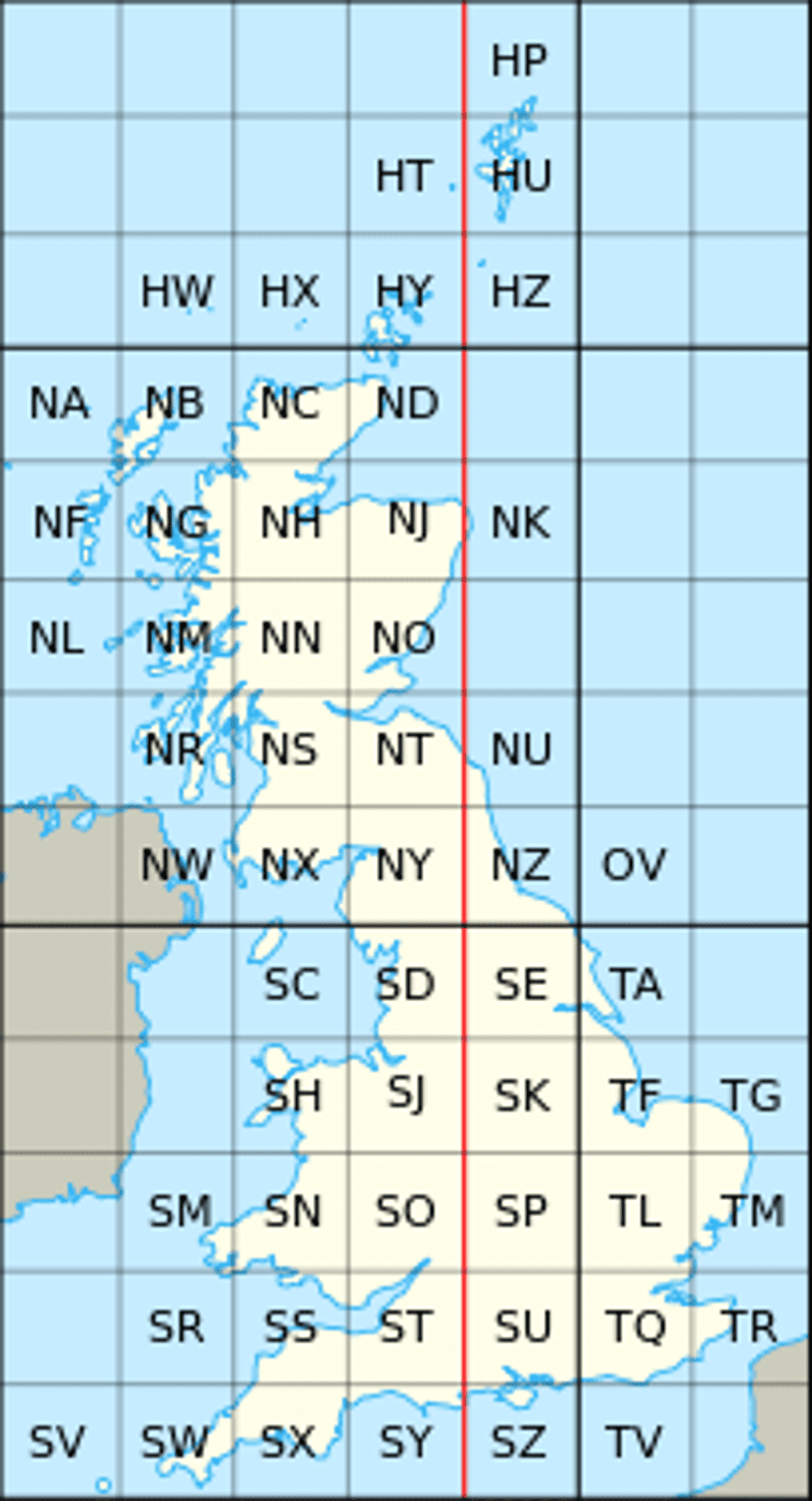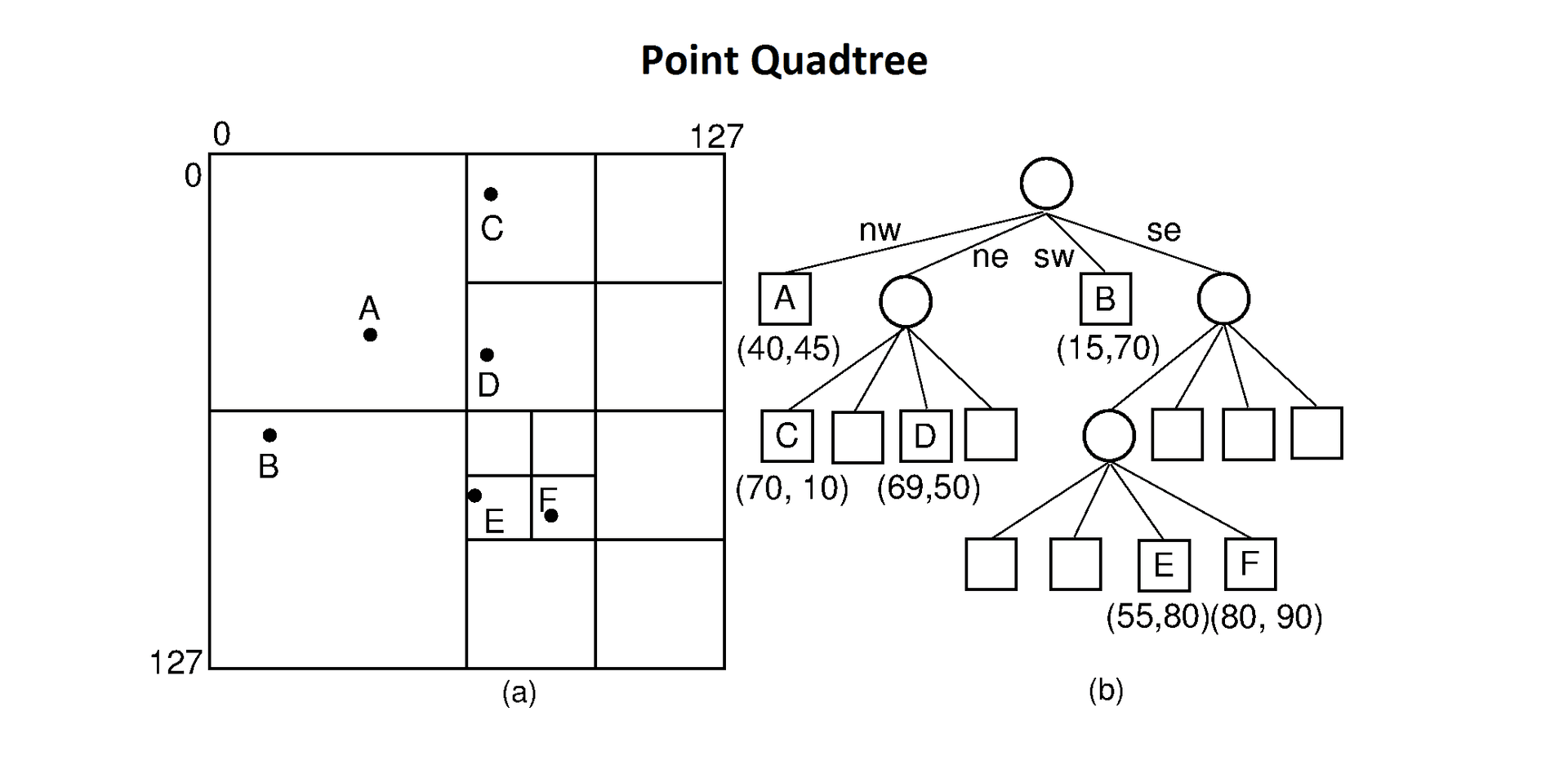# The Hard Thing About Hard Geo Things

As spatial data sets have become larger, aggregated faster and from a wider variety of sources, handling large spatial datasets has become a challenge. As I'm sure many of you have experienced, many GIS operations can take a fairly long time to complete, especially with traditional desktop GIS techniques. Last week at the pub after geomob, I was discussing with a friend who works for Google in the Maps team about how they deal with such large datasets, and what techniques you can use to solve problems with them.

• What sort of computational complexity are we looking at for fundamental GIS operations?
• What problems can be parallelized or are suitable for approaches such as MapReduce?For the purpose of this post we will use Big O notation to express the complexity of a problem. Big O notation expresses how complex a problem in relation to its inputs. This allows it to be neutral of all other factors such as hardware, data size (i.e. MB, GB etc), time units and so forth. As an example you can see in the graph above a linear problem (green - O(n)) requires a lot less operations to complete than a quadratic problem (light blue - O(n2)).

At its most basic level a geocode could be seen as a database lookup; the user searches for a postcode, this is checked in a database and a corresponding latitude and longitude is returned. The problem is slightly more complex than this obviously, but such a geocoder would without any optimisations be a O(n) problem. A O(n) problem is a linear problem who's number of operations to complete is matched by the number of inputs given to it (i.e. 100 inputs results in 100 operations). This problem actually becomes a O(log n) problem if we were to index the postcode column.

Another example of a linear GIS problem is a geographic transformation (scale, rotate, translation etc) as we loop through all coordinates (inputs) and perform some mathematical function to transform the coordinates position. Similarly in a coordinate system reprojection we are mapping a function against all coordinates to transform the coordinate position into another coordinate system. As we only loop through the data and operate on it once, the problem is again linear.

However, let's take an example problem to work out which UK postcodes belong to which Ordnance Survey grid cell. In human terms this a trivial problem, as one can deduct very quickly and visually which postcode centroid is in which cell.However for a machine that problem is slightly more complex. It needs to programmatically deduce which grid cell it is in. This problem is actually a point in bounding box problem, which is a slightly simpler derivative of the point in polygon (PIP) problem. Ignoring this function for a minute however, we examine that in order to solve the problem we must loop through all 1.8 million UK postcodes and determine which of the 55 Ordnance Survey grid cells this sits in!

A naive pseudocode implementation might look like:

``	for postcode in postcodes:		for grid in osgrid:			if pointInBoundingBox(postcode, grid):				return True``

The double for loop inherently makes this a O(n * m) problem which in worst case scenario a O(n2) problem i.e. n and m are of equal length. Ignoring Big O notation for a moment, we can actually say specifically for this problem it will take 99,000,000 operations to complete. This doesn't take into account the added complexity that might be added by the internals of the pointInBoundingBox function (more for loops and so forth). Essentially, to obtain the answer we must compare both sets of inputs against each other which can lead to some very large number of operations as they increase. Let's just say don't be too surprised when your desktop GIS crashes solving these kinds of problems!

A slightly more nuanced approach might use some sort of R-Tree/Quadtree/etc to allow for a better way of dividing up and searching through the grid cells and postcode points. These data structures allow data to be better searched as we don't have to loop through all of the inputs only the parent (containing) cells.Unfortunately the process of building the tree is essentially a very similar problem to the original question itself. To build the tree you have to loop through all the points and all the grid cells which is of equal complexity to solving the original question. A quote I remember from my old job regarding this situation is to solve the problem, you have to solve the problem.

Spatial indexes on spatial databases use such trees to speed up spatial querying. Using spatial index and subsequently a data structure to quickly determine which section of a coordinate space a geometry exists in allows for faster querying. Here you save a lot of time on the queries (for reasons expressed above) but indexing all the data in the first place will take time in itself.

The next question is could we parallelize this problem? That is to say, can we split this problem into smaller chunks and process it across multiple cores or machines? We can, but only if don't divide the OS grid cells. Let me explain why; let's say we use half the grid cells to one machine along with half the points to be processed. Because we know the points must have the correct grid cells to pass the test, we can't just send any grid cells and any points to the machines. In order to find out which grid cell a point resides in we have no option but to test against all the grid cells (in the naive approach). However, we can divvy up the postcode points in any way we want to. This whole process isn't too bad as we only have 55 grid cells in this example, but let's say we had a million grid cells, you can see how the computational time rapidly increases.

As you can see the problem is inherently complex due to the multidimensional nature of geographic data and the kinds of topological questions we ask of it. Alongside this most problems in GIS revolve around at least two data sets, and often these can be very large in size. Many operations, for example the topological query explained above require a function to be performed that requires looping through the datasets. You can see another example of a O(n2) algorithm comparing line intersection algorithms here: Wise 2014. Indeed many of these types of problems are around the O(n2) complexity mark for the worst case, with the 2 increasing upwards as you increase the number of datasets in the operation. Not only that but many of the operations have the issue that because the spatial dimension of the data is key to solving the operation, we can't actually split the data up anyway we might like.

Above this, certain routing algorithms can be of O(n3) that use heuristics (rules of thumb) to find good solutions (Fischer et al, 2009). Using bruteforce (testing all solutions) routing algorithms can be as bad as O(cn) where c is a positive constant. In the most naive approach the algorithm is problem is actually O(n!) (i.e. the factorial of the number of inputs).

Obviously there are many problems that are much harder than the geo problems expressed above, for example writing a program that can beat a human at a game of Go, which has 10 101023 possible moves. However hopefully this post has nonetheless illustrated the complexities with handling big geo datasets. It has been demonstrated that although all problems vary in complexity, many of our day to day GIS operations that involve two data sets are of approximately O(n2) complexity without optimisations, and are also tricky to parallelize.

I would be really interested in hearing people's insights as to how the industry/academia is moving forward in solving these problems. Equally if you have any corrections or points of contentions please feel free to reach out.

Published